# Triangle Angle Calculator

Created by Hanna Pamuła, PhD candidate
Reviewed by Bogna Szyk
Last updated: Jun 20, 2022

Triangle angle calculator is a safe bet if you want to know how to find the angle of a triangle. Whether you have three sides of a triangle given, two sides and an angle or just two angles, this tool is a solution to your geometry problems. Below you'll also find the explanation of fundamental laws concerning triangle angles: triangle angle sum theorem, triangle exterior angle theorem, and angle bisector theorem. Read on to understand how the calculator works, and give it a go - finding missing angles in triangles has never been easier!

## How to find the angle of a triangle

There are several ways to find the angles in a triangle, depending on what is given: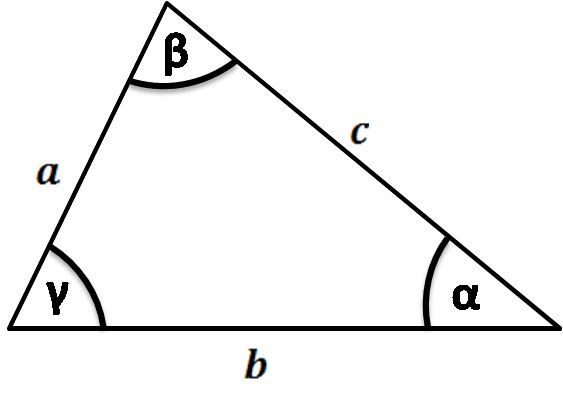1. Given three triangle sides

Use the formulas transformed from the law of cosines:

• cos(α) = (b² + c² - a²)/ 2bc,

so α = arccos [(b² + c² - a²)/(2bc)]

• cos(β) = (a² + c² - b²)/ 2ac,

so β = arccos [(a² + c² - b²)/(2ac)]

• cos(γ) = (a² + b² - c²)/ 2ab,

so γ = arccos [(a² + b² - c²)/(2ab)]

1. Given two triangle sides and one angle

If the angle is between the given sides, you can directly use the law of cosines to find the unknown third side, and then use the formulas above to find the missing angles, e.g. given a,b,γ:

• calculate c = √[a² + b² - 2ab * cos(γ)]
• substitute c in α = arccos [(b² + c² - a²)/(2bc)]
• then find β from triangle angle sum theorem: β = 180°- α - γ

If the angle isn't between the given sides, you can use the law of sines. For example, assume that we know a, b, α:

• a / sin(α) = b / sin(β) so β = arcsin[b * sin(α) / a]
• As you know, the sum of angles in a triangle is equal to 180°. From this theorem we can find the missing angle: γ = 180°- α - β
1. Given two angles

That's the easiest option. Simply use the triangle angle sum theorem to find the missing angle:

• α = 180°- β - γ
• β = 180°- α - γ
• γ = 180°- α - β

In all three cases, you can use our triangle angle calculator - you won't be disappointed.

## Sum of angles in a triangle - Triangle angle sum theorem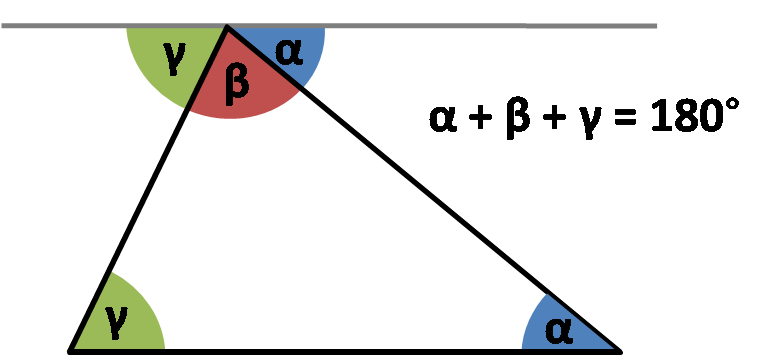The theorem states that interior angles of a triangle add to 180°:

α + β + γ = 180°

How do we know that? Look at the picture: the angles denoted with the same Greek letters are congruent because they are alternate interior angles. Sum of three angles α, β, γ is equal to 180°, as they form a straight line. But hey, these are three interior angles in a triangle! That's why α + β + γ = 180°.

## Exterior angles of a triangle - Triangle exterior angle theorem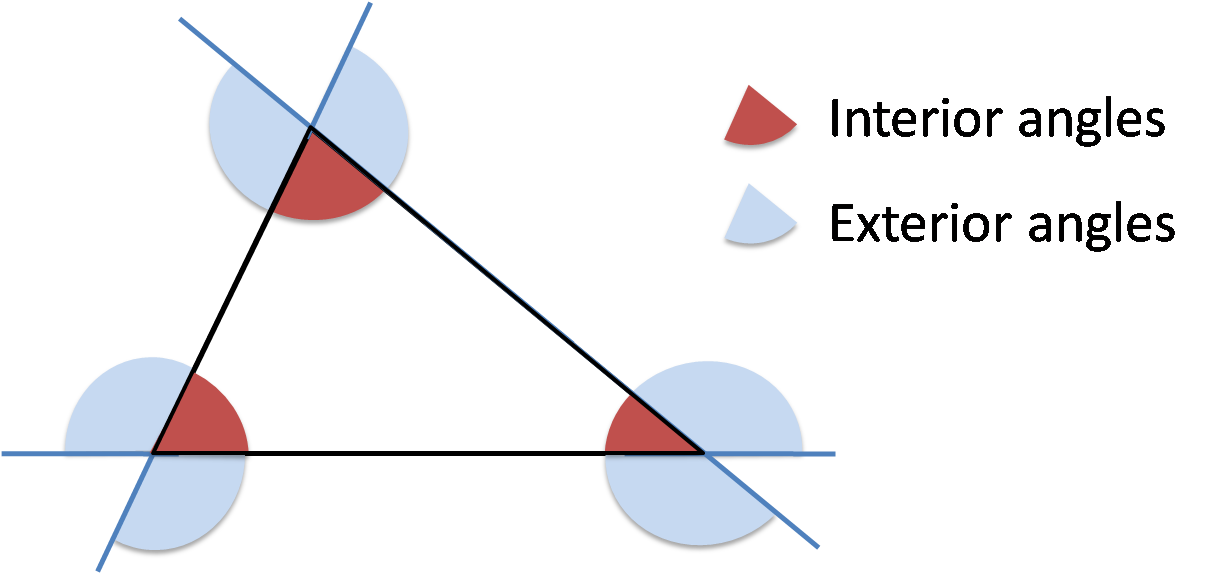An exterior angle of a triangle is equal to the sum of the opposite interior angles.

• Every triangle has six exterior angles (two at each vertex are equal in measure).
• The exterior angles, taken one at each vertex, always sum up to 360°.
• An exterior angle is supplementary to its adjacent triangle interior angle.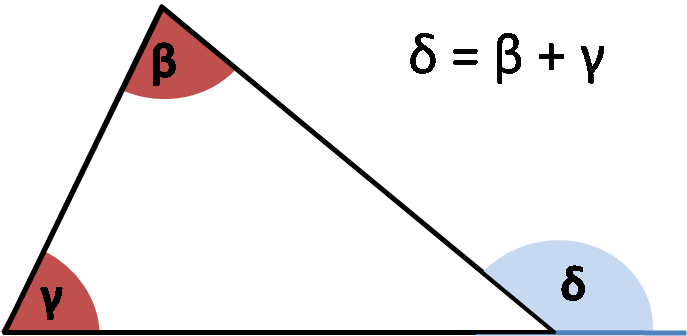## Angle bisector of a triangle - Angle bisector theorem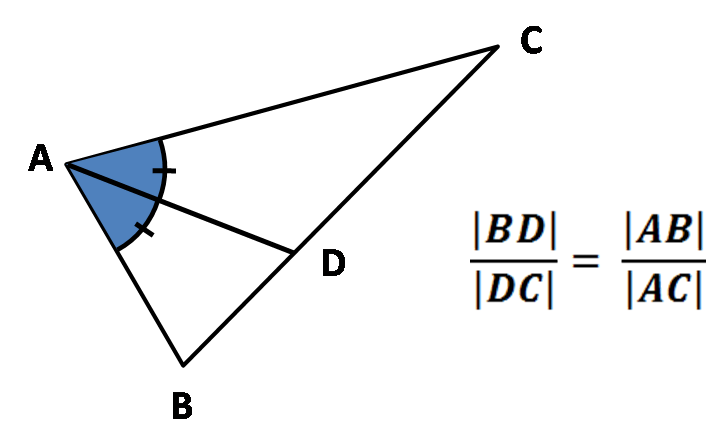Angle bisector theorem states that:

An angle bisector of a triangle angle divides the opposite side into two segments that are proportional to the other two triangle sides.

Or, in other words:

The ratio of the BD length to the DC length is equal to the ratio of the length of side AB to the length of side AC:

|BD|/|DC|= |AB|/|AC|

## Finding missing angles in triangles - example

OK, so let's practice what we just read. Assume we want to find the missing angles in our triangle. How to do that?

1. Find out which formulas you need to use. In our example, we have two sides and one angle given. Choose angle and 2 sides option.
2. Type in the given values. For example, we know that a = 9 in, b = 14 in and α = 30°. If you want to calculate it manually, use law of sines:
• a / sin(α) = b / sin(β), so

β = arcsin[b * sin(α) / a] =

arcsin[14 in * sin(30°) / 9 in] =

arcsin[7/9] = 51.06°

• From the theorem about sum of angles in a triangle, we calculate that γ = 180°- α - β = 180°- 30° - 51.06° = 98.94°

1. The triangle angle calculator finds the missing angles in triangle. They are equal to the ones we calculated manually: β = 51.06°, γ = 98.94°; additionally, the tool determined the last side length: c = 17.78 in.

## FAQ

### How do I find angles in a triangle?

To determine the missing angle(s) in a triangle, you can call upon the following math theorems:

• The fact that the sum of angles is a triangle is always 180°;
• The law of cosines; and
• The law of sines.

### Which set of angles can form a triangle?

Every set of three angles that add up to 180° can form a triangle. This is the only restriction when it comes to building a triangle from a given set of angles.

### Why can't a triangle have more than one obtuse angle?

This is because the sum of angles in a triangle is always equal to 180°, while an obtuse angle has more than 90° degrees. If you had two or more obtuse angles, their sum would exceed 180° and so they couldn't form a triangle. For the same reason, a triangle can't have more than one right angle!

### How do I find angles of the 3 4 5 triangle?

Let's denote a = 5, b = 4, c = 3.

1. Write down the law of cosines 5² = 3² + 4² - 2×3×4×cos(α). Rearrange it to find α, which is α = arccos(0) = 90°.
2. You can repeat the above calculation to get the other two angles.
3. Alternatively, as we know we have a right triangle, we have b/a = sin β and c/a = sin γ.
4. Either way, we obtain β ≈ 53.13° and γ ≈ 36.87.
5. We quickly verify that the sum of angles we got equals 180°`, as expected.
Hanna Pamuła, PhD candidate
Given
angle and 2 sides
Angle selection
αα
deg
a
in
b
in
c
in
β
deg
γ
deg
People also viewed…

### Bitwise

Use the bitwise calculator to execute bitwise AND, OR, and XOR on inputs from different numeral systems.

### Car vs. Bike

Everyone knows that biking is awesome, but only this Car vs. Bike Calculator turns biking hours into trees! 🌳

### Chilled drink

With the chilled drink calculator you can quickly check how long you need to keep your drink in the fridge or another cold place to have it at its optimal temperature. You can follow how the temperature changes with time with our interactive graph.

### Circumcenter of a triangle

With the circumcenter calculator you'll discover how to use the coordinates of a triangle's vertices to get the coordinates of the circumcenter.